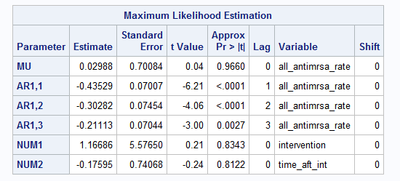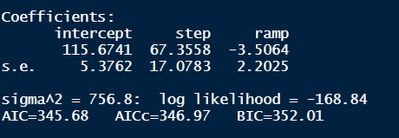## ARIMA model to proc ARIMA

Hi,

I am working on an intervention/ITS analysis. I first ran the analysis in R and would like to marginally replicate my results in SAS but I am having trouble. Given my ARIMA model from R and current proc arima code, I was hoping that you could point me in the right direction as the results do not make sense.

R model ARIMA=(3,0,0)

proc arima data=one;

identify=y cross=(step ramp);

estimate p=3 input=(step ramp) method=ml outmodel=out;

run;

results

SASRThank you!

1 ACCEPTED SOLUTION

Accepted Solutions

## Re: ARIMA model to proc ARIMA

hello @Levi_M

I do not have knowledge about R code or output to know exactly the equation that is being fit in your R code to compare with your PROC ARIMA code. So I can only comment on what your PROC ARIMA code is doing, it is an ARIMA(3,0,0) model with two input series (which is a regression with AR(3) error). The model is estimated using maximum likelihood estimation method in your code with method = ML option. The mathematical equation for the ARIMA(3,0,0) model with two input series in your PROC ARIMA is below:

Y_t = mu + w1*X1_t + w2*X2_t +  1/ (1-phi1*B-phi2*B^2-phi3*B^3)  *a_t

You may also check the following section of PROC ARIMA documentation for discussions of regression with ARMA errors, and the mathematical equation specified, for similar regression models with ARMA errors in general:

https://go.documentation.sas.com/doc/en/pgmsascdc/v_030/etsug/etsug_arima_gettingstarted25.htm#etsug...

The example in the doc above is ARIMA(1,0,1) with two inputs, different than the one you specified, but it should give you an example what equation is being fit when regression with ARMA error is fit in PROC ARIMA in general.

You may want to verify the equation being fit in your R code and make sure the equation fit are the same before you make any comparison.

2 REPLIES 2

## Re: ARIMA model to proc ARIMA

hello @Levi_M

I do not have knowledge about R code or output to know exactly the equation that is being fit in your R code to compare with your PROC ARIMA code. So I can only comment on what your PROC ARIMA code is doing, it is an ARIMA(3,0,0) model with two input series (which is a regression with AR(3) error). The model is estimated using maximum likelihood estimation method in your code with method = ML option. The mathematical equation for the ARIMA(3,0,0) model with two input series in your PROC ARIMA is below:

Y_t = mu + w1*X1_t + w2*X2_t +  1/ (1-phi1*B-phi2*B^2-phi3*B^3)  *a_t

You may also check the following section of PROC ARIMA documentation for discussions of regression with ARMA errors, and the mathematical equation specified, for similar regression models with ARMA errors in general:

https://go.documentation.sas.com/doc/en/pgmsascdc/v_030/etsug/etsug_arima_gettingstarted25.htm#etsug...

The example in the doc above is ARIMA(1,0,1) with two inputs, different than the one you specified, but it should give you an example what equation is being fit when regression with ARMA error is fit in PROC ARIMA in general.

You may want to verify the equation being fit in your R code and make sure the equation fit are the same before you make any comparison.

## Re: ARIMA model to proc ARIMA

Thank you for your feedback. Unfortunately, I do not know R very well either and a package was using fit parameters ie AIC to determine the model without providing much context. However, the resources you provide helped me continue working on my project.

Thank you.

Discussion stats
• 2 replies
• 1125 views
• 0 likes
• 2 in conversation